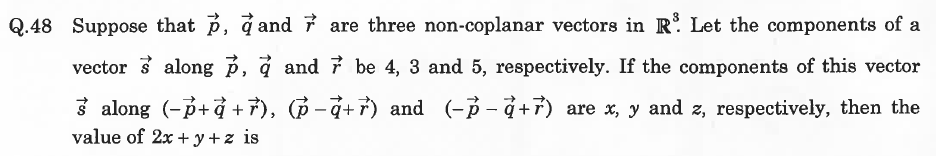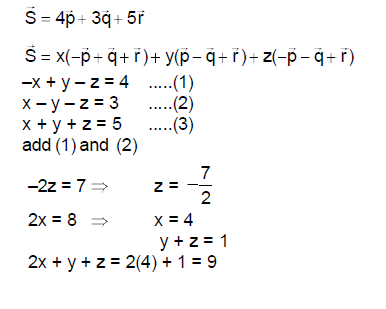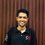# JEE Advanced 2015- Incorrect problem, open discussion.If you can in any way contribute, please do.

The above problem appeared in the JEE- Advanced 2015 examination in the second paper. The answer provided in the official answer key is $9$. However, the problem is widely believed to be incorrect. The authorities conducting the examination accept suggestions related to the correctness of the published key till 11- Jun 2015, 12 noon (Indian standard time). I want to send them a fax related to the error in this problem and to do so, it would really help if the community could offer valuable suggestions about the problem.

Here is an (incorrect) solution which yields the supposed answer:Note by Raghav Vaidyanathan
6 years ago

This discussion board is a place to discuss our Daily Challenges and the math and science related to those challenges. Explanations are more than just a solution — they should explain the steps and thinking strategies that you used to obtain the solution. Comments should further the discussion of math and science.

When posting on Brilliant:

• Use the emojis to react to an explanation, whether you're congratulating a job well done , or just really confused .
• Ask specific questions about the challenge or the steps in somebody's explanation. Well-posed questions can add a lot to the discussion, but posting "I don't understand!" doesn't help anyone.
• Try to contribute something new to the discussion, whether it is an extension, generalization or other idea related to the challenge.
• Stay on topic — we're all here to learn more about math and science, not to hear about your favorite get-rich-quick scheme or current world events.

MarkdownAppears as
*italics* or _italics_ italics
**bold** or __bold__ bold
- bulleted- list
• bulleted
• list
1. numbered2. list
1. numbered
2. list
Note: you must add a full line of space before and after lists for them to show up correctly
paragraph 1paragraph 2

paragraph 1

paragraph 2

[example link](https://brilliant.org)example link
> This is a quote
This is a quote
    # I indented these lines
# 4 spaces, and now they show
# up as a code block.

print "hello world"
# I indented these lines
# 4 spaces, and now they show
# up as a code block.

print "hello world"
MathAppears as
Remember to wrap math in $$ ... $$ or $ ... $ to ensure proper formatting.
2 \times 3 $2 \times 3$
2^{34} $2^{34}$
a_{i-1} $a_{i-1}$
\frac{2}{3} $\frac{2}{3}$
\sqrt{2} $\sqrt{2}$
\sum_{i=1}^3 $\sum_{i=1}^3$
\sin \theta $\sin \theta$
\boxed{123} $\boxed{123}$

## Comments

Sort by:

Top Newest

If you think a solution is wrong, always work on finding out what the potential issue is, and why you disagree with it. In the case, since the solution appears correct / has no mistakes, then you should ask "How can this problem yield multiple answers"?

If you cannot convince yourself that there should be multiple/different answers, nor justify why this seemingly correct solution is wrong, then it is most likely correct. IE The burden of proof is on you.

The misconception that you have is in thinking that "the components of this vector are obtained from the projection down to the individual axis". This statement is only true if the axis are orthogonal to each other, like in the $i j k$ case that we're used to.

As an easy (counter)example, think about the point $(1, 1, 0)$ and the axis of $(1,0,0), (0,1,0), (1, 1, 1)$. Clearly, the projections onto these axis are $(1,0,0), (0,1,0)$ and something non-zero.

However, the components of $(1,1, 0)$ is not " $(1,0,0) + (0,1,0) +$ something non-zero".

Staff - 6 years ago

Log in to reply

Thanks for all your help. The final answer key was released today after considering feedback from public. It has been declared that all candidates be given marks for this particular problem regardless of the answer they have marked.

- 6 years ago

Log in to reply

- 6 years ago

Log in to reply

Th answer provided is absolute incorrect.

- 6 years ago

Log in to reply

Thanks for your comment sir. I just wanted to make sure that it was indeed incorrect before sending them a suggestion.

- 6 years ago

Log in to reply

A little thing you can add in your suggestion in against of the answer is :

If we take $\vec{p}=\hat{i},\vec{q}=\hat{j},\vec{r}=\hat{k}$, then $\vec{s}=4\hat{i}+3\hat{j}+5\hat{k}$ and now the question can be solved easily. and the answer comes out to be $4\sqrt{3}$ (hope I made no calculation errors) :P

- 6 years ago

Log in to reply

Yes, you are right. I marked $4$ as the answer due to this reason. Although now I feel like $7$ might have been a better choice.

- 6 years ago

Log in to reply

×

Problem Loading...

Note Loading...

Set Loading...